Capitol College

Spring Semester 2000

# Circuit Theory: EE - 159

Lab Experiment #3

## Mesh Analysis Verification

Andy Buettner

Instructor: Dr. Thomas

Due: March 21st, 2000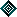Objective

The objective of this lab is to determine the currents in a circuit with multiple sources using mesh analysis. Then, use mesh analysis to analyze a complex circuit. Lastly to compare the results with experimentally determined values.Equipment Used

1. Equipment used

1. ET - 3100 Trainer #1517

2. Fluke DMM ser. no: 31101554

3. Lab kit EE - 159

1. Components used

1. 470 Ohm Resistor

2. 680 Ohm Resistor

3. 330 Ohm Resistor

4. 1K Ohm Resistor

5. 220 Ohm ResistorProcedures

### I) Part 1

1) Measure and record the individual resistances of each resistor

2) Assemble circuit from Diagram 1

3) Set voltages for both sides of the source

4) Measure voltages across each resistor

1. #### Part 2

1) Measure the individual resistances of the resistors

2) Assemble the circuit in diagram 2

3) Set voltage on Trainer to 10 volts

4) Measure the voltages across each resistorResultsTable 1

R1

R2

##### R3

R4

###### Measured Resistance

459 W

670 W

329 W

990 W

Measured Voltage

1.838V

2.685V

.510V

5.47V

Calculated Current

4.004ma

4.007ma

1.550ma

5.525ma

Determined Current

3.994ma

3.994ma

1.551ma

5.545ma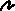Table 2

 R1 R2 R3 R4 R5 Measured Resistance 459 W 670 W 329 W 990 W 218 W Measured Voltage 6.16V 2.960V 3.483V 6.48V 3.811V Calculated Current 13.42ma 4.418ma 10.59ma 6.545ma 17.48ma Determined Current 13.47ma 4.03ma 10.61ma 6.58ma 17.50ma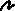Diagram 1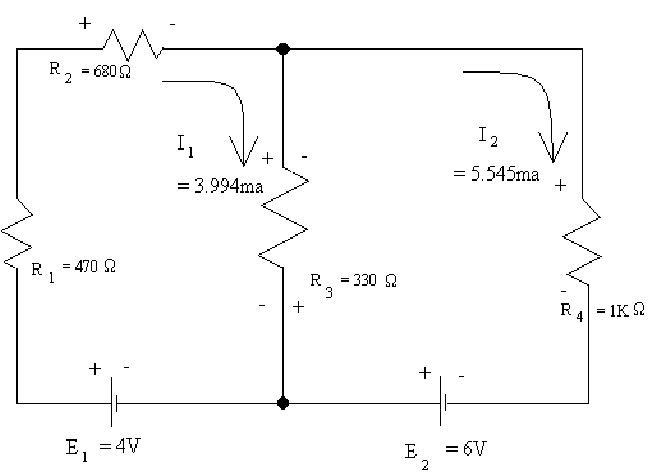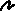Diagram 2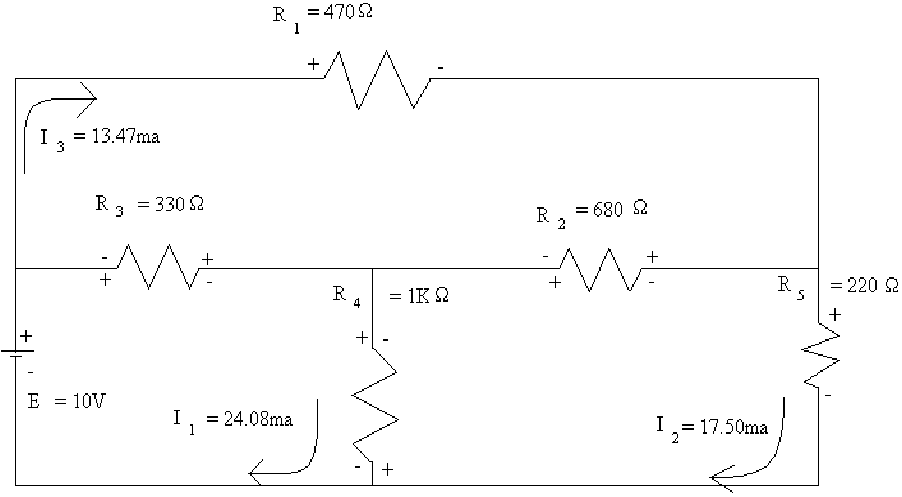Answers to Lab Questions

1. Q: Calculate the currents through each resistor using mesh analysis.

A: Answers in tables 1 & 2.

2) Q: Calculate the currents through each resistor using Ohm's law.

A: Answers in Tables 1 & 2.

3) Q: Compare results.

A: The results are nearly identical and in some cases are.

4) Q: Explain how a negative voltage can be made to be positive.

A: Reverse the poles of the volt - meter.

5) Q: The trainer can only produce differences of 15V. How can a difference of 30V be obtained?

A: By using both the positive and negative terminals instead of the ground terminal.6) Q: What does the DMM read when the battery is low?

A: There is an icon in the top center that shows a battery with an X through it.Conclusions

In order to complete this lab I used several aids to help me. First, to completely analyze the mesh analysis, I used my equation-solving program to perform the matrix math. Also, I used the found values of the resistors instead of the read ones to complete the mesh analysis. I found it interesting to find out exactly how little error was involved in this exercise.Attachments

1. Original Handout

2. Original Data

3. Calculations